/

### Extreme Events – Specimen Question A.5.2(a) – Answer/Hints

Q. You know that she has also used mean-variance optimisation techniques and adopted the same expected covariances as you would have done in A.5.1. What return assumptions might she have adopted when choosing her portfolio mix? Specify mathematically all possible sets of return assumptions she could have adopted and still reached this answer.

The return assumptions that your colleague might have used can be derived using implied alphas. These are the (mean) returns that your colleague needs to have assumed will apply for the portfolio in question to be deemed efficient.

No constraints are biting in relation to the specific portfolio mix in question (apart from the constraint that all weights add to unity). If returns assumed for each asset category are characterised by the vectorand if the portfolio weights are characterised by the vector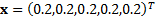then we need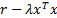to be at a maximum, subject to the constraint that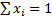. Using Lagrange multipliers as per Section 5.10 of Extreme Events, this implies that: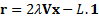It is relatively straightforward to calculate an examplethat satisfies this equation using Microsoft Excel. Alternatively, you could use the Nematrian web function, MnReverseQuadraticPortfolioOptimiser, which assumes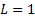but allows an arbitrary choice of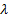(the input parameter is there called the ‘TradeOffFactor’). Choosing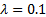gives the following possible values for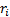:1 (i.e. A1) -0.288 2 (i.e. A2) -0.864 3 (i.e. A3) 2.656 4 (i.e. A4) 5.18 5 (i.e. A5) 6.48